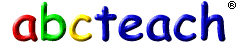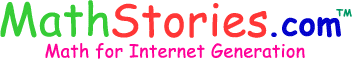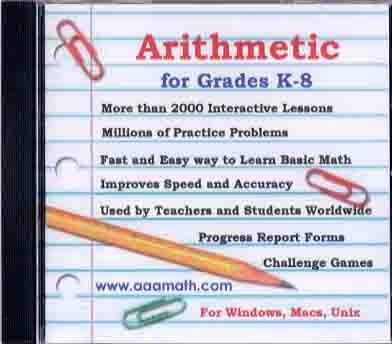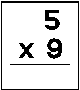Lots of games to practice all kinds of second grade math.Take a train ride to see how well you can do!

Math Baseball
Test your mathskills while scoring some runs!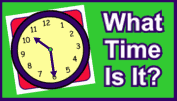Let's Tell Time
Practice telling time while playing this game

\$\$\$  Money Flashcards \$\$\$

\$\$\$   Money Flashcards \$\$\$\$\$
Practice counting money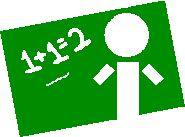The ArithmAttack
A great way to practice those math facts. You have 60 seconds to complete as many facts as you can. A great site to visit often to keep up those math facts.Soft Schools.com

First and second Grade Math worksheets and GamesVisit the sites below to practice math skills by playing FUN games!Numbers and Patterns •  Place Value Puzzler •  Dragon Eggs (Odd/Even) •  Least to Greatest Practice •  Number Cracker (Patterns) •  Pattern Mania •  Pattern Quest Time •  Willy the Watch Dog (half hour) •  Stop the Clock •  Bang On Time •  Telling Time •  What Time Will It Be? •  Set the Clock •  Feeding Time •  Teaching Clock Money •  Piggy Bank •  Counting Change •  Counting Money •  Counting Money 2 •  Spending Spree •  Coin Values Problem Solving •  Math Puzzles - Genuis Tests •  Mental Math •  Math Cats Story Problems •  Math Cats Story Problems 2 Addition & Subtraction •  Addition Matho •  Addition Machine •  Number Monster •  The Timernator •  Subtraction Machine •  Subtraction Hidden Picture •  Math Baseball •  Sum Sense •  High Rise Math (2 Players) •  Subtraction Tic Tac Toe (2 Players) Multiplication •  Multiplication Hidden Picture •  Are You a Math Magician? •  Sum Sense (Multiplication) Graphing, Data, and Probability •  Saxon Math Graphing •  Create A Graph •  Tally Charts •  Bar Graph Creator •  Graph Goodies •  Probability •  Mean, Median, and Mode •  Interpreting Data Geometry •  Shape Sorter •  Shape Transformations •  Tangrams •  Triangle Sort •  Quadrilateral Sort •  Shape Riddles •  3D Shape Match •  Polygon Playground •  Angle Sort •  Lines of Symmetry Game Fractions •  Bowling for Fractions •  Pizza Party (Fractions) •  Fraction Race •  Fraction Concentration •  Cross the River Measurement •  Adam Ant Perimeter •  Measure It! •  Can You Fill It? •  Virtual Ruler Other Resources •  Virtual Manipulatives •  Houghton Mifflin Brain Teasers •  Houghton Mifflin Test Prep